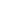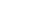How Cheenta works to ensure student success?
Explore the Back-Story

# Test of Mathematics Solution Subjective 57 - Number of Six Letter WordsThis is a Test of Mathematics Solution Subjective 57 (from ISI Entrance). The book, Test of Mathematics at 10+2 Level is Published by East West Press. This problem book is indispensable for the preparation of I.S.I. B.Stat and B.Math Entrance.

Also visit: I.S.I. & C.M.I. Entrance Course of Cheenta

## Problem

How many 6-letter words can be formed using the letters A,B and C so that each letter appears at least once in the word.

## Solution:

Number of ways 6 letter words can be formed using letters A,B,C so that each letter appears at least once in the word = Number of ways 6 letter word formed using A,B,C - Number of ways 6 letter word formed using any two of A,B,C + Number of 6 letter words formed by using A,B,C. [ using inclusion exclusion principle. ]

=-+= 555 [ans]This is a Test of Mathematics Solution Subjective 57 (from ISI Entrance). The book, Test of Mathematics at 10+2 Level is Published by East West Press. This problem book is indispensable for the preparation of I.S.I. B.Stat and B.Math Entrance.

Also visit: I.S.I. & C.M.I. Entrance Course of Cheenta

## Problem

How many 6-letter words can be formed using the letters A,B and C so that each letter appears at least once in the word.

## Solution:

Number of ways 6 letter words can be formed using letters A,B,C so that each letter appears at least once in the word = Number of ways 6 letter word formed using A,B,C - Number of ways 6 letter word formed using any two of A,B,C + Number of 6 letter words formed by using A,B,C. [ using inclusion exclusion principle. ]

=-+= 555 [ans]

### Knowledge Partner